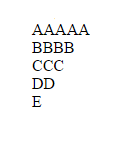# How to print alphabet series in php

We can make alphabet pyramid series very easily using PHP. To print this pyramid pattern in php, we can use different types of method.

In first program we are using range function which store values in an array from A to Z.
Now we use two ‘FOR’ loops. In first ‘for’ loop we run condition from 1 to 5 to print 5 rows, and in second ‘for’ loop we set conditions in decreasing order to print our objects. And the value of \$i will responsible for the output. i.e.
If \$i = 0 then \$letter[‘0’] = A, \$i=1 then \$letter[‘1’] = B and so on.

 ``` \$i; \$j--){ echo \$letters[\$i]; } echo "
"; } ?> ```Method:2
In method second we use chr() function to get the output in character. The ASCII value of A,B,C,D,E is 65,66,67,68,69.
The chr() function return the value of ASCII code i.e.
chr(65) = A, chr(66) = B and so on.
The working of both the ‘for’ loops is same as above method.

 ``` =\$i-64; \$j--){ echo chr(\$i); } echo "
"; } ?> ```
Method: 3
In third method we again use range() function from A to Z. But here we use ‘foreach’ loop along it.
The range function contain values from A to Z in an array and the ‘foreach’ loop return all values one by one with \$char variable. Here we use a single ‘for’ loop to print the output in a periodic method.

 ```=\$k; \$i--){ echo \$char; } echo "
"; \$k=\$k+1; } ?> ```